# please tell me if its a z test ke t test and why it is :)...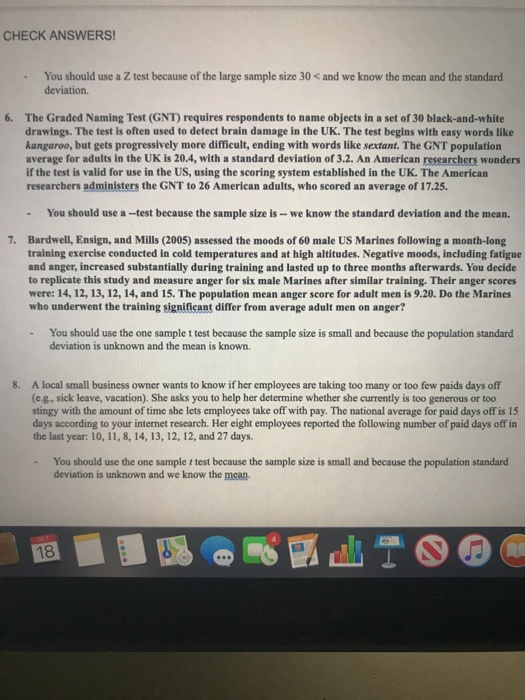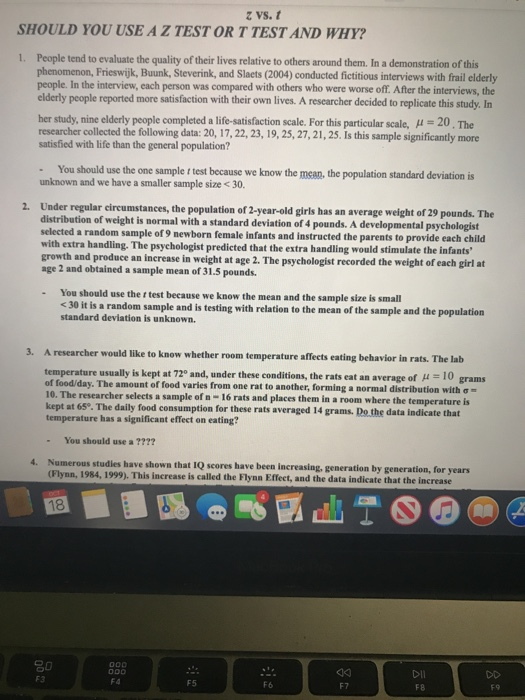please tell me if its a z test ke t test and why it is :) thanks!
CHECK ANSWERS! You should use a Z test because of the large sample size 30 < and we know the mean and the standard deviation. 6. The Graded Naming Test (GNT) requires respondents to name objects in a set of 30 black-and-white drawings. The test is often used to detect brain damage in the UK. The test begins with easy words like kangaroo, but gets progressively more difficult, ending with words like sextant. The GNT population average for adults in the UK is 20.4, with a standard deviation of 3.2. An American researchers wonders if the test is valid for use in the US, using the scoring system established in the UK. The American researchers administers the GNT to 26 American adults, who scored an average of 17.25. - You should use a-test because the sample size is -- we know the standard deviation and the mean. 7. Bardwell, Ensign, and Mills (2005) assessed the moods of 60 male US Marines following a month-long training exercise conducted in cold temperatures and at high altitudes. Negative moods, including fatigue and anger, increased substantially during training and lasted up to three months afterwards. You decide to replicate this study and measure anger for six male Marines after similar training. Their anger scores were: 14, 12, 13, 12, 14, and 15. The population mean anger score for adult men is 9.20. Do the Marines who underwent the training significant differ from average adult men on anger? . You should use the one sample t test because the sample size is small and because the population standard deviation is unknown and the mean is known. 8. A local small business owner wants to know if her employees are taking too many or too few paids days off (e.g., sick leave, vacation). She asks you to help her determine whether she currently is too generous or too stingy with the amount of time she lets employees take off with pay. The national average for paid days off is 15 days according to your internet research. Her eight employees reported the following number of paid days off in the last year: 10, 11, 8, 14, 13, 12, 12, and 27 days. You should use the one sample i test because the sample size is small and because the population standard deviation is unknown and we know the mean.
z vs. t SHOULD YOU USE A Z TEST OR T TEST AND WHY? 1. People tend to evaluate the quality of their lives relative to others around them. In a demonstration of this phenomenon, Frieswijk, Buunk, Steverink, and Slaets (2004) conducted fictitious interviews with frail elderly people. In the interview, each person was compared with others who were worse off. After the interviews, the elderly people reported more satisfaction with their own lives. A researcher decided to replicate this study. In her study, nine elderly people completed a life-satisfaction scale. For this particular scale, 4=20. The researcher collected the following data: 20, 17, 22, 23, 19, 25, 27, 21, 25. Is this sample significantly more satisfied with life than the general population? You should use the one sample t test because we know the mean, the population standard deviation is unknown and we have a smaller sample size < 30 2. Under regular circumstances, the population of 2-year-old girls has an average weight of 29 pounds. The distribution of weight is normal with a standard deviation of 4 pounds. A developmental psychologist selected a random sample of 9 newborn female infants and instructed the parents to provide each child with extra handling. The psychologist predicted that the extra handling would stimulate the infants growth and produce an increase in weight at age 2. The psychologist recorded the weight of each girl at age 2 and obtained a sample mean of 31.5 pounds. You should use the r test because we know the mean and the sample size is small <30 it is a random sample and is testing with relation to the mean of the sample and the population standard deviation is unknown. 3. A researcher would like to know whether room temperature affects eating behavior in rats. The lab temperature usually is kept at 720 and, under these conditions, the rats eat an average of 10 grams of food/day. The amount of food varies from one rat to another, forming a normal distribution with e 10. The researcher selects a sample of n- 16 rats and places them in a room where the temperature is kept at 65°. The daily food consumption for these rats averaged 14 grams. Do the data indicate that temperature has a significant effect on eating? You should use a ???? 4. Numerous studies have shown that IQ scores have been increasing, generation by generation, for years (Flynn, 1984, 1999). This increase is called the Flynn Effect, and the data indicate that the increase 18 o00 000 DII DD F3 F4 F6 E7 F8

When we use Z-test and T-test:-

When population standard deviation () is known, then we use Z- test and if population standard deviation () is unknown, then we use t- test.

1. You should use one sample t test because here mean () is given but population standard deviation () is unknown.

2. You should use One sample Z- test because here mean () is given but population standard deviation () is also known.

3. You should use One sample Z- test because here mean () is given but population standard deviation () is also known.

6. You should use One sample Z- test because here mean () is given but population standard deviation () is also known.

7. You should use one sample t test because here mean () is given but population standard deviation () is unknown.

8. You should use one sample t test beause here mean () is given but population standard deviation () is unknown.

#### Earn Coin

Coins can be redeemed for fabulous gifts.

Similar Homework Help Questions

PLEASE HELP! Z-test or T-test? Give a full explanation please! A researcher wants to compare the email use of the employees at one company to the corresponding population. Data is collected on the number of emails received by the company's employees. It will be necessary to use this sample data, as well as population data to determine if there is a significant difference between both. Population Mean number of emails: 25 Standard deviation: 3 Sample Mean number of emails: 20...

• ### A psychologist has developed an aptitude test which consists of a series of mathematical and vocabulary...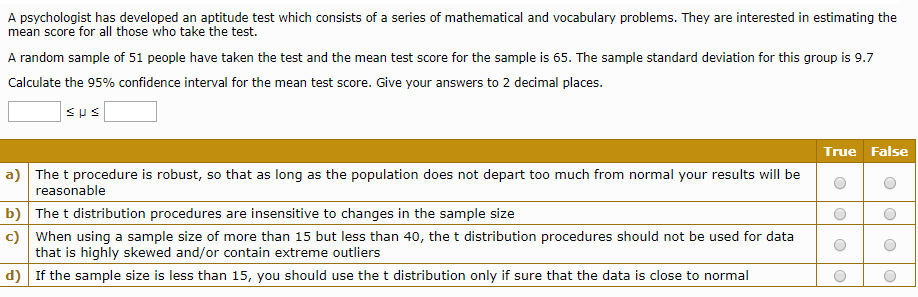A psychologist has developed an aptitude test which consists of a series of mathematical and vocabulary problems. They are interested in estimating the mean score for all those who take the test. A random sample of 51 people have taken the test and the mean test score for the sample is 65. The sample standard deviation for this group is 9.7 Calculate the 95% confidence interval for the mean test score. Give your answers to 2 decimal places. True False...

• ### 1) Timothy has finished calculating a z-test. Now he turns to the table of z-scores to...

1) Timothy has finished calculating a z-test. Now he turns to the table of z-scores to find the: a) Critical value b) Test value c) calculated value d) obtained value 2) Joyce has calculated a Z-test with an alpha set to .05. She calculates a z-value of 6.36. What can she conclude? a) none of these b) reject the null hypothesis c) fail to reject the null hypothesis d) the result is too close to call 3) In order to...

• ### z-test a sample for the null hypothesis using α = 0.05 as our threshold a) Given...

z-test a sample for the null hypothesis using α = 0.05 as our threshold a) Given a population with mean of 550 and variance of 100, what would be minimum sample mean to reject the null hypothesis if our sample size is 20? b) Given a population with mean of 550 and variance of 100, what would be maximum sample mean to reject the null hypothesis if our sample size is 20? c) Let’s assume the same population mean and...

• ### Suppose that we want to estimate the mean germination time of strawberry seeds. The germination times...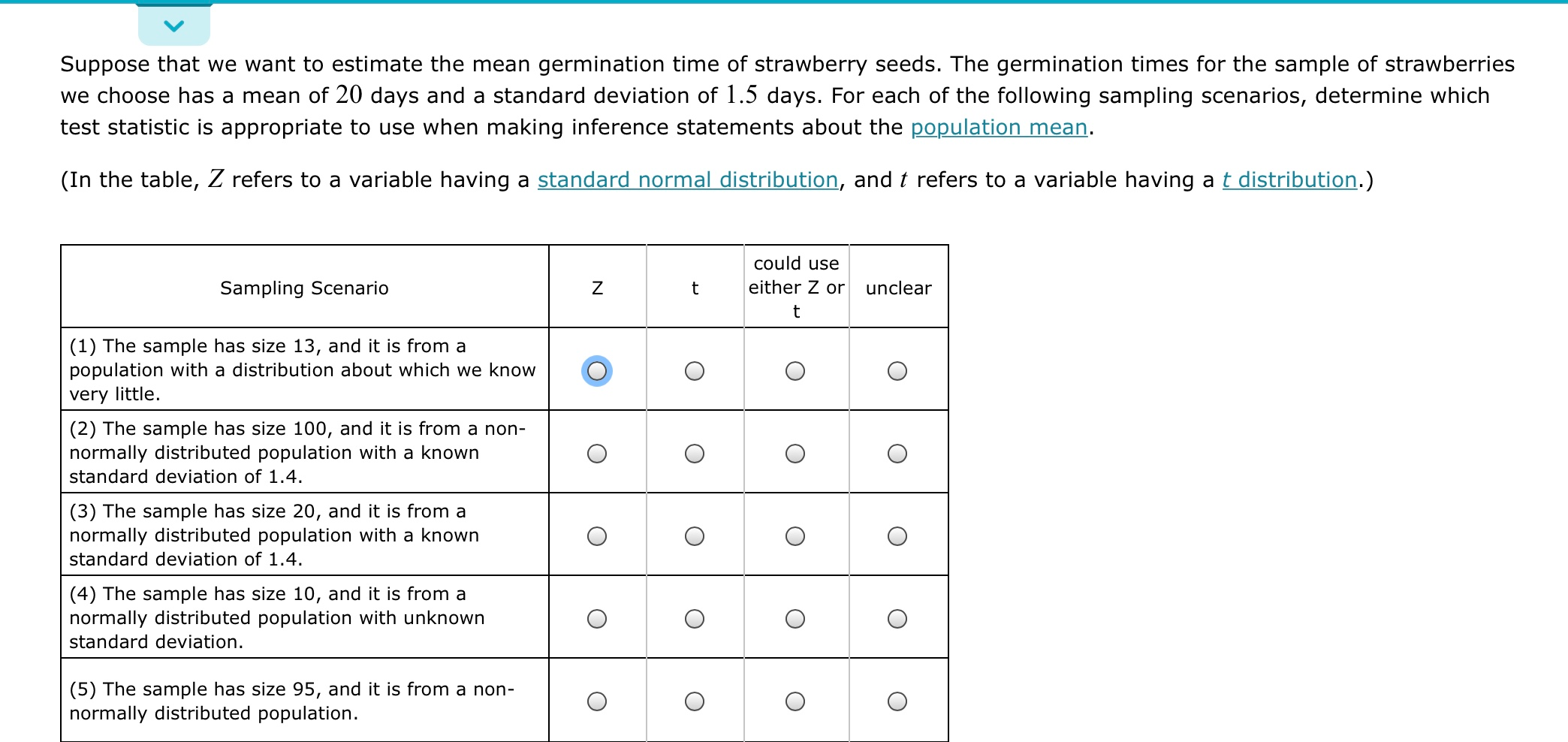Suppose that we want to estimate the mean germination time of strawberry seeds. The germination times for the sample of strawberries we choose has a mean of 20 days and a standard deviation of 1.5 days. For each of the following sampling scenarios, determine which test statistic is appropriate to use when making inference statements about the population mean. (In the table, Z refers to a variable having a standard normal distribution, and t refers to a variable having a...

• ### Question Which of the following is true about the one sample z-test and one sample t-test:...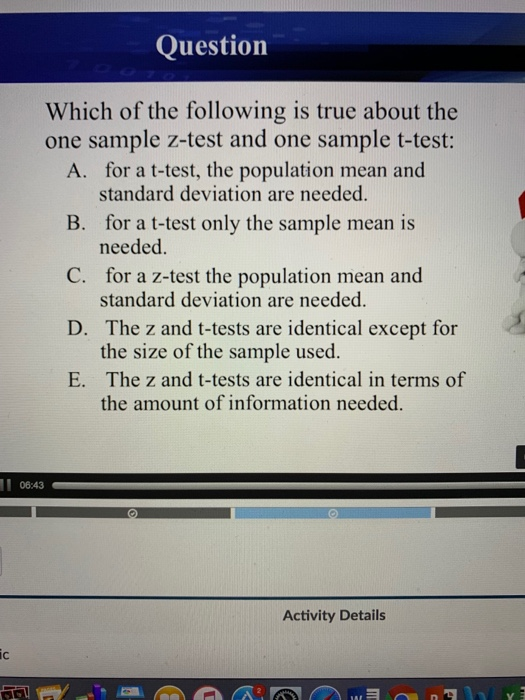Question Which of the following is true about the one sample z-test and one sample t-test: A. for a t-test, the population mean and standard deviation are needed for a t-test only the sample mean is needed. for a z-test the population mean and standard deviation are needed. The z and t-tests are identical except for the size of the sample used. The z and t-tests are identical in terms of the amount of information needed. B. C. D. E....

• ### Q3. Hypothesis Testing with a Z Test (12 points) According to the CDC report, the mean...

Q3. Hypothesis Testing with a Z Test (12 points) According to the CDC report, the mean life expectancy in the US population is currently (µ) 78.6 years with a standard deviation (σ) of 12. A researcher examined the age of death of 400 people recently recorded in several Arizona hospitals and calculated the mean to be 79.5 years old. He runs a two-tailed Z test with α = .05 to see if Arizona has a significantly different life expectancy compared...

• ### Imagine that a researcher appropriately conducts a z test and then calculates a corresponding 95% confidence...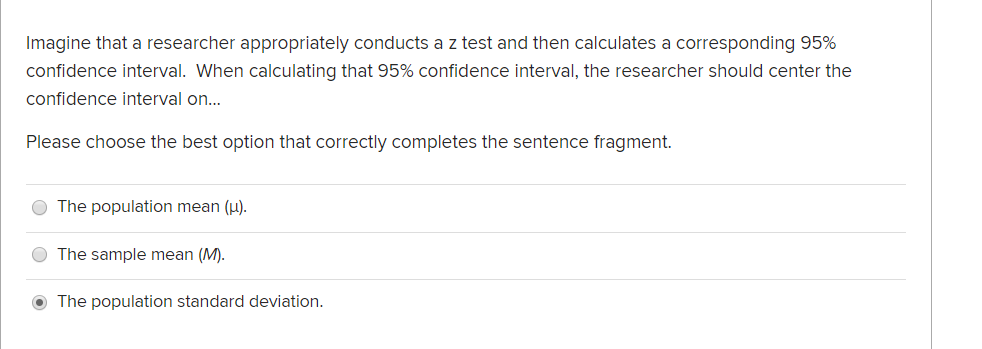Imagine that a researcher appropriately conducts a z test and then calculates a corresponding 95% confidence interval. When calculating that 95% confidence interval, the researcher should center the confidence interval on... Please choose the best option that correctly completes the sentence fragment. The population mean (u). The sample mean (M). The population standard deviation.

• ### If we know the population standard deviation and we use the t-test instead of the Z-test,...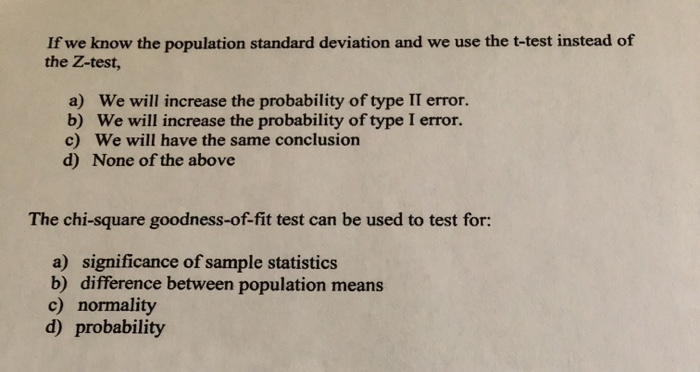If we know the population standard deviation and we use the t-test instead of the Z-test, a) We will increase the probability of type II error. b) We will increase the probability of type I error. c) We will have the same conclusion d) None of the above The chi-square goodness-of-fit test can be used to test for: a) significance of sample statistics b) difference between population means c) normality d) probability

• ### quiz 6

1.A researcher is interested in determining whether the color of an animal's surroundings affects learning rate and tests 16 rats in a box with colorful wallpaper.Assume that it is known that the average rat can learn to run this particular maze (in a box without any special coloring) in 25 trials, with a variance of 64, andthat the distribution is normal. The mean number of trials to learn the maze for the group with the colorful wallpaper is 11. What...